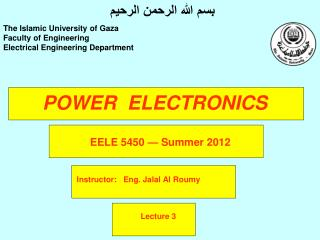DownloadDownload PresentationPOWER ELECTRONICS

# POWER ELECTRONICS

Download Presentation## POWER ELECTRONICS

- - - - - - - - - - - - - - - - - - - - - - - - - - - E N D - - - - - - - - - - - - - - - - - - - - - - - - - - -
##### Presentation Transcript

1. بسم الله الرحمن الرحيم The Islamic University of Gaza Faculty of Engineering Electrical Engineering Department POWER ELECTRONICS EELE 5450— Summer 2012 Instructor: Eng. Jalal Al Roumy Lecture 3

2. Chapter Two Power Semiconductor Diodes Power diodes have large power-, voltage-, current-handling capabilities than that of ordinary signal diodes.

3. Chapter Two Power Diodes symbol and type of packaging

4. Chapter Two Diode Characteristics

5. Chapter Two • V-I characteristics • Forward-biased region • Reverse biased region • Breakdown region

6. Chapter Two Shockley’s equation N=1,2( Germanium, Silicon) practically 1.1—1.8 VT= Thermal voltage IS= Saturation current

7. Chapter Two Shockley diode equation

8. Chapter Two Forward-bias VD>0

9. Chapter Two Reverse-bias VD<0

10. Chapter Two Reverse Recovery Characteristics Tb/Ta is the softness factor (SF)

11. Chapter Two Power Diode Types • Ideally, a diode should have no reverse recovery time, However, the manufacturing cost of such diode will increase. • The power diodes can be classified into three categories: • Standard or general-purpose diodes • Fast-recovery diodes • Schottky diodes

12. Chapter Two Standard or general-purpose diodes • Reverse recovery time= 25 us (relatively high). • Used in Low-speed applications. • Current rating : From less than 1 Ampere to several thousands of Amperes. • Voltage rating 50 v to 5 kV.

13. Chapter Two Fast-Recovery Diodes • Reverse recovery time< 5 us ( Low). • Used in DC-DC and DC-AC converter circuit (high speed applications). • Current rating : From less than 1 Ampere to several hundreds of Amperes. • Voltage rating 50 v to 3 kV. • Platinum or gold.

14. Chapter Two SchottkyDiodes • No minority carriers . • Recovered charge is much less than P-n junction diodes. • Used in high current and low voltage dc power supplies. • Current rating : From 1 Ampere to 300 Amperes. • Voltage rating <100 V.

15. Chapter Two • The reverse recovery time of a diode is trr=3us and the rate of fall of the diode current is di/dt=30 A/us • Determine • (a) the storage charge Qrr • (b) Peak reverse current Irr End of Lecture